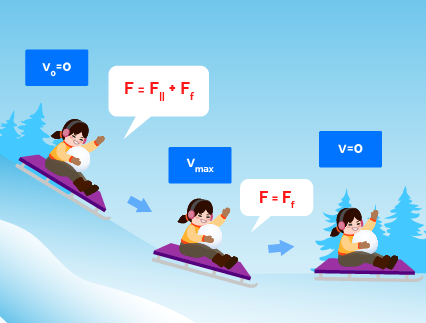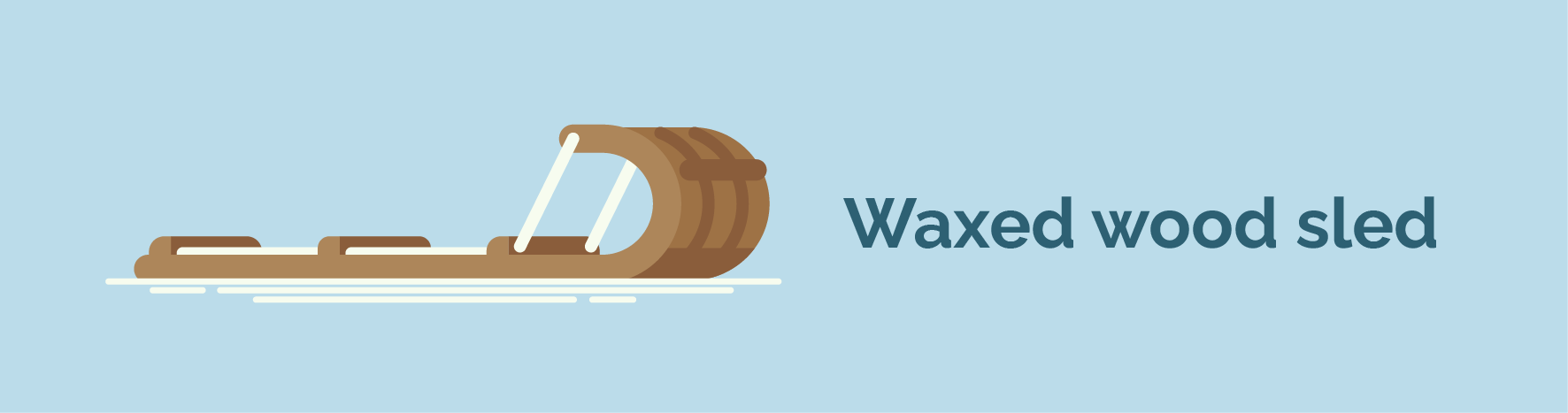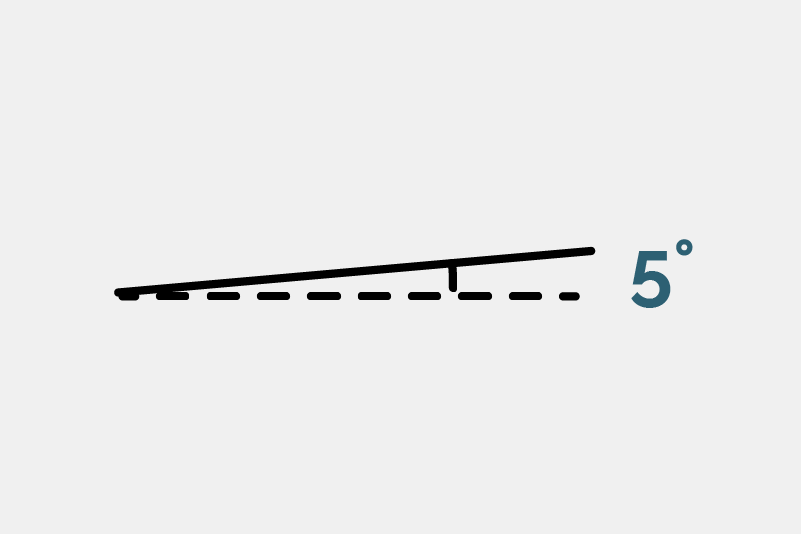# Sled Ride Calculator

Created by Davide Borchia
Reviewed by Bogna Szyk
Last updated: Feb 15, 2022

Snowy days are the best part of winter! Bring your children out in the snow to enjoy it (or enjoy it yourself 😉), but be safe and use our sled calculator to check if a slope is A-OK to sled on. Many injuries happen every year, mostly due to careless practices — that's why we're here to help!

Sledding in particular can be dangerous, but a little bit of science can help you decide if the hill you are looking at is safe or not. If you give us a couple of measurements 📏, our calculator will tell you:

• The maximum speed reached by your kid; and
• How far they will go after the slope has levelled out.

We are going to give you a couple of directions, but in the end, trust your judgement!

Why did we build this calculator? As scientists, we can't help but looking at numbers — they are everywhere — and the statistics for sledding accidents are scary! ! So we asked ourselves: How can we change those numbers, with the help of numbers?

When you are done with sledding, or if you are looking for a more relaxing activity, why not build a snowman? We have the perfect tool for that: try our snowman calculator!

## Sledding day!

Did it snow tonight? Has the storm calmed a little? Brace yourself, wear thick gloves and warm clothes, and prepare to spend a day on the snow pulling sleds to the top of the slope. But maybe check that your kid is not projected into orbit at the end of their descent!

Sleds have always been one of the best ways to move on ice and snow! Think of skis, but without the instability and the uncomfortable boots. Also, they can be easily pulled: dogs, horses, and parents are the preferred choices for this.

Sledding is tons of fun, as everything regarding snow tends to be. But, like every activity involving some kind of action, it may be dangerous.

🔎 Both the North Pole and the South Pole were reached by expeditions using sledges. Amundsen, the Norwegian explorer, made the crucial decision to use dogs during the expedition against Robert Scott, even though the ethics behind their treatment is... !

Let's be honest about it, without beating around the bush. Every year, around 20 thousand kids end up with some injuries due to sledding, in the US alone. It's a pretty huge number! Most of the injuries are fractures (and they are nasty to heal, trust us, especially if the kid loves the outdoors), and they mainly affect kids between the ages of 10 and 14. But following a couple of safety rules, you can help lower these figures, while keeping your kid (and yourself) safe!

Most of the injuries happen due to collisions, and speed is a crucial factor here! Follow the next steps to find out the speed of your kid during the descent.

## What’s the physics behind our sled ride calculator?

It all boils down to an inclined plane problem — with friction!

When the sled slides down the slope, two forces act on it: gravity and friction. Let's analyse them:

• Gravity is defined as $F_g = m g$, where $m$ is the mass of the object (in this case the sled plus the kid) times the gravitational acceleration $g = 9.81\ \text{m}/\text{s}^2$.
• Friction is the product of the normal force $F_N$ (the reaction to the weight of an object) times $\mu$, the friction coefficient: $F_f = \mu F_N$.

The force of gravity can be separated into two components, one perpendicular and one parallel to the slope's plane. They depend on the angle $\theta$ at which the plane is tilted. Here they are:

• The parallel component is equal to $F_{\Vert}=m g \sin{\left(\theta\right)}$. It's the only one controlling the speed of the object.
• Perpendicular component: $F_{⊥}=m g \cos{\left(\theta\right)}$. In a frictionless plane, this component would not matter at all, but in presence of friction, it equals the normal force $F_N$.

💡 Drag due to air resistance is a factor too, but it can be easily ignored at the speed your kid will go down the slope. Think of riding a bike. You won't feel the wind if you keep a moderate pace. Try to race a little, and you will find that tucking behind the handlebar will help you go even faster.

The acceleration of the system sled plus kid is given by the sum of the forces acting on it:

$\begin{split} F_\text{total} &= F_\Vert + F_f \\ &= F_\Vert + \mu F_⊥ \\ &= mg\sin(\theta) + \mu mg \cos(\theta) \end{split}$

Now, we would like to introduce Isaac Newton, whose second principle states that force is equal to mass times acceleration, or $F = m a$.

Let's write the total force this way, and look:

$m a = m g \sin{\left(\theta\right)} + \mu m g\cos{\left(\theta\right)}$

The mass appears on both sides of the equation, and we can cancel it out:

$a = g \sin{\left(\theta\right)} + \mu g \cos{\left(\theta\right)}$

This is the acceleration! The fact that it doesn't depend on the mass is one thing us physicists will never get tired of — it's so counterintuitive, but Galileo didn't drop those weights for nothing!

🔎 The mass doesn't matter in the ideal, world where there's no air resistance, cows are spherical, and so on. Astronauts on the Moon dropped , at the same time, and they landed together! In the real world, it does matter: massive objects usually pack more weight for the same value of surface area, and are more effective against air resistance. In the case of sledding down a slope, it's not a big problem, though!

The second part of the problem is located in the flat space after the slope has leveled out. In this part, the acceleration due to gravity is not present anymore, and only the friction acts on the sled. Now the normal force is not the perpendicular component anymore, but it takes all of the gravity force since $\theta = 0$ and $\sin{\left(\theta\right)} = 0$ and $\cos{\left(\theta\right)} = 1$. The friction is then $F_f = \mu m g$, and the total acceleration (which in this case is obviously a deceleration) is:

$\begin{split} m a &= \mu m g \\ a &= \mu g \end{split}$

## How to use the sled ride calculator?

First choose the type of sled you are using. This is extremely important! The type of material, and the quality of the snow affect the ride, by a lot. For example, metal goes faster than every other common material in use for sledges. Teflon (the polymer used in non-stick pans) is a runner-up, but is not really common!

As for the snow, sledding on fresh, fluffy snow is not the best for a fast ride. Snow that is compact and a little wet makes for the fastest descents.

It's all a matter of friction: if you want to learn more about it, try our friction calculator.

💡 The best way to know if a snowy day is optimal for sledding is by checking the thermometer: below −5 degrees Celsius (20 F), the snow will be too light. Around 0 degrees Celsius (30 F) is the best temperature for a memorable day. And if you need help to switch from Fahrenheit to Celsius, we got your back with our temperature converter!

You need to measure the slope you are going to use. It sounds difficult, but we swear, you won't need a measurement tape or anything else, you are going to be your own instrument.

1. Measure the slope's length. You can use any GPS app, or you can count your steps. To do this, determine your step length. Now walk down the slope, counting your steps, and then multiply the number of steps you've just taken with your step length to obtain the slope's length. Easy peasy!
2. Measure the angle. This is a little bit more complicated. Check our graphics below, or use an app that allows you to simulate a protractor on your phone.

💡 Estimating angles is hard. This is even more true when you are close to the slope, or you don't have a clear view. Try to guess the best angle of your slope, it's important!
In the calculator, you will find some selected values and a graphic for each one of them; choose the best fitting one.

The motion of your kid on their sledge is what physicists call uniformly accelerated motion. Lucky for us, there is a nice set of equations that can give you everything you need to know about the motion of an object with just a few initial values. Let's sketch them out!

We use these variables in the equations:

• $x$ — the position of the sledge;
• $v$ — the velocity of the sledge;
• $a$ — the acceleration of the sledge;
• $t$ — the time at which we look at the sledge; and
• $\ell$ — the length of the slope.

We call the initial position and velocity $x_0$ and $v_0$ respectively. In our calculations, we will assume both of these values to be $0$, as we're starting from rest on the top of the slope.

At any given time $t$, the speed of the sledge is:

$v_t = a t + v_0$

and its position is:

$x = x_0 + v_0 t + \frac{1}{2}a t^2$

🙋 We ask you to input those value in order to find the acceleration of the sled during the descent. That's all we need to do all the math!

Now, to find the final speed down the slope, we just reverse the equation. We've already found the acceleration $a = g \sin{\left(\theta\right)} + \mu g \cos{\left(\theta\right)}$. Now we can find the time it takes to slide down the slope, $t_\text{slope}$. Ignoring the initial speed and position (as they're both $0$), we have:

$t_\text{slope} = \sqrt{\frac{2\ell}{a}}$

Now it is possible to calculate the speed of the sledge at the bottom of the slope:

$v_\text{bottom} = a t_\text{slope}$How the total force and the speed vary during a descent.

⚠️ Sleds can easily reach speeds higher than 40 km/h (25 mph) — that's a lot! We decided to give you a warning if the expected speed reaches this value.

The second part of the problem is again a uniformly accelerated motion. To be precise, we told you, we should say "decelerated", but it all boils down to a minus sign!

The equations are the same, apart from the fact that now the initial speed $v_0$ equals the top speed at the end of the descent, i.e. $v_\text{bottom}$. From that value, and with the deceleration due to friction, we can easily find the length and the distance covered by the sled before it stops.

🔎 We decided to show you only the top speed and the distance covered after the slope. The other quantities we wrote about are "hidden" in the advanced mode. Click on that button and explore our calculator a little bit more!

## How to be safer?

If you are a worrying parent, put a helmet on your kid's head. But also remember that humans did without it for centuries. As for everything, just use common sense: don't let your child sled close to cars or trees.

It's a good idea to teach your kid some basic rules:

• Always ride feet first!
• Don't use the sled with more people than it's built for.
• If your kid wears a scarf, be sure everything is tucked in (why not a neck warmer then?).
• If possible, choose a steerable sled with brakes!
• Check what's after the slope: preferably nothing, and be careful to avoid the presence of roads and buildings.

⚠️ Science is good, but your attention is always needed! Use our tool as an indication, but always think before pushing your kid down a slope! Choose the right precautions, in every situation — but don't isolate your kid in a danger-free world! Let them go out and play.

## A final message

The day is over — the sunset came and went and it's already dark! We hope you had a safe and entertaining day on the snow, alone, with your kids or whoever you love. Now go get something warm — maybe in front of a fireplace!

Davide Borchia
Did you wake up to a thick blanket of snow ❄? It may be the perfect day for sledding 🛷! Check if the hill you and your kids are going to sled on is safe with our calculator. A little bit of science can help keeping one of the best days of the winter from a bad turn.
Hill shape and sled type
Type of sled
Waxed wood on dry snowHill length
ft
Hill angleSliding down the slope
How fast will they go at the bottom?
mph
Sliding time
sec
Traveling after the slope
Distance to stop
ft
Time to stop
sec
People also viewed…

### Factor of Safety

The factor of safety calculator obtains the ratio of an object's or structure's maximum strength to its design load, to determine how safe it is. Read on to learn more about factor of safety and its applications.

### Normal force

Find out the normal force value of an object on a flat and inclined surface with our normal force calculator.